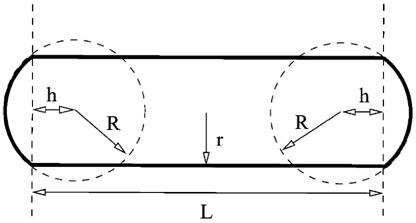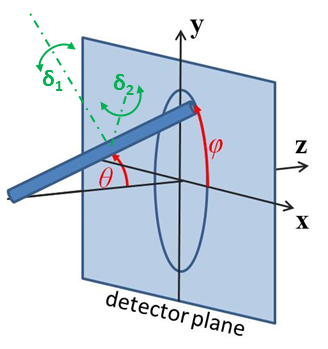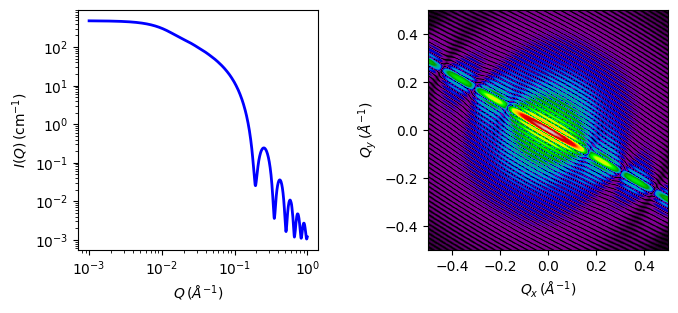# capped_cylinder

Right circular cylinder with spherical end caps and uniform SLD

Parameter

Description

Units

Default value

scale

Scale factor or Volume fraction

None

1

background

Source background

cm-1

0.001

sld

Cylinder scattering length density

10-6-2

4

sld_solvent

Solvent scattering length density

10-6-2

1

20

20

length

Cylinder length

400

theta

cylinder axis to beam angle

degree

60

phi

degree

60

The returned value is scaled to units of cm-1 sr-1, absolute scale.

Definitions

Calculates the scattering from a cylinder with spherical section end-caps. Like barbell, this is a sphereocylinder with end caps that have a radius larger than that of the cylinder, but with the center of the end cap radius lying within the cylinder. This model simply becomes a convex lens when the length of the cylinder $$L=0$$. See the diagram for the details of the geometry and restrictions on parameter values.Fig. 4 Capped cylinder geometry, where $$r$$ is radius, $$R$$ is radius_cap and $$L$$ is length. Since the end cap radius $$R \geq r$$ and by definition for this geometry $$h \le 0$$, $$h$$ is then defined by $$r$$ and $$R$$ as $$h = -\sqrt{R^2 - r^2}$$

The scattered intensity $$I(q)$$ is calculated as

$I(q) = \frac{\Delta \rho^2}{V} \left<A^2(q,\alpha).sin(\alpha)\right>$

where the amplitude $$A(q,\alpha)$$ with the rod axis at angle $$\alpha$$ to $$q$$ is given as

$\begin{split}A(q) =&\ \pi r^2L \frac{\sin\left(\tfrac12 qL\cos\alpha\right)} {\tfrac12 qL\cos\alpha} \frac{2 J_1(qr\sin\alpha)}{qr\sin\alpha} \\ &\ + 4 \pi R^3 \int_{-h/R}^1 dt \cos\left[ q\cos\alpha \left(Rt + h + {\tfrac12} L\right)\right] \times (1-t^2) \frac{J_1\left[qR\sin\alpha \left(1-t^2\right)^{1/2}\right]} {qR\sin\alpha \left(1-t^2\right)^{1/2}}\end{split}$

The $$\left<\ldots\right>$$ brackets denote an average of the structure over all orientations. $$\left< A^2(q)\right>$$ is then the form factor, $$P(q)$$. The scale factor is equivalent to the volume fraction of cylinders, each of volume, $$V$$. Contrast $$\Delta\rho$$ is the difference of scattering length densities of the cylinder and the surrounding solvent.

The volume of the capped cylinder is (with $$h$$ as a positive value here)

$V = \pi r_c^2 L + 2\pi\left(\tfrac23R^3 + R^2h - \tfrac13h^3\right)$

and its radius of gyration is

$\begin{split}R_g^2 =&\ \left[ \tfrac{12}{5}R^5 + R^4\left(6h+\tfrac32 L\right) + R^3\left(4h^2 + L^2 + 4Lh\right) + R^2\left(3Lh^2 + \tfrac32 L^2h\right) \right. \\ &\ \left. + \tfrac25 h^5 - \tfrac12 Lh^4 - \tfrac12 L^2h^3 + \tfrac14 L^3r^2 + \tfrac32 Lr^4 \right] \left( 4R^3 + 6R^2h - 2h^3 + 3r^2L \right)^{-1}\end{split}$

Note

The requirement that $$R \geq r$$ is not enforced in the model! It is up to you to restrict this during analysis.

The 2D scattering intensity is calculated similar to the 2D cylinder model.Fig. 5 Definition of the angles for oriented 2D cylinders.Fig. 6 1D and 2D plots corresponding to the default parameters of the model.

Source

capped_cylinder.py $$\ \star\$$ capped_cylinder.c $$\ \star\$$ gauss76.c $$\ \star\$$ sas_J1.c $$\ \star\$$ polevl.c

References

1. H Kaya, J. Appl. Cryst., 37 (2004) 223-230

2. H Kaya and N R deSouza, J. Appl. Cryst., 37 (2004) 508-509 (addenda and errata)

1. Onsager, Ann. New York Acad. Sci., 51 (1949) 627-659

Authorship and Verification

• Author: NIST IGOR/DANSE Date: pre 2010

• Last Modified by: Paul Butler Date: September 30, 2016

• Last Reviewed by: Richard Heenan Date: January 4, 2017